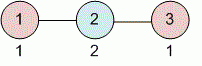Competitions

# Degrees of vertices

A simple undirected graph is given with adjacency matrix. Find the degrees of all its vertices.

#### Input

The first line contains the number of vertices in a graph n (1n100). Each of the next n lines contains n elements - the description of adjacency matrix.

#### Output

Print n integers - the degrees of all vertices.Time limit 1 second
Memory limit 128 MiB
Input example #1
3
0 1 0
1 0 1
0 1 0

Output example #1
1
2
1